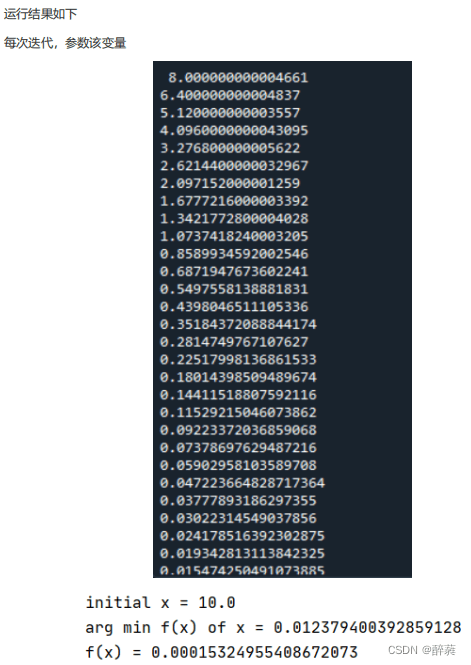# 一、 什么是梯度下降算法

首先，我们需要明确梯度下降就是求一个函数的最小值，对应的梯度上升就是求函数最大值。简而言之：梯度下降的目的就是求函数的极小值点，例如在最小化损失函数或是线性回归学习中都要用到梯度下降算法。

##梯度下降算法作为很多算法的一个关键环节，其重要意义是不言而喻的。

梯度下降算法的思想：先任取点（x0,f(x0))，求f(x)在该点x0的导数f"(x0),在用x0减去导数值f"(x0),计算所得就是新的点x1。然后再用x1减去f"(x1)得x2…以此类推，循环多次，慢慢x值就无限接近极小值点。

损失函数用来衡量机器学习模型的精确度。一般来说，损失函数的值越小，模型的精确度就越高。 如果要提高机器学习模型的精确度，就需要尽可能降低损失函数的值。而降低损失函数的值，我们 一般采用梯度下降这个方法。所以，梯度下降的目的，就是为了最小化损失函数。

梯度下降法的基本思想可以类比为一个下山的过程。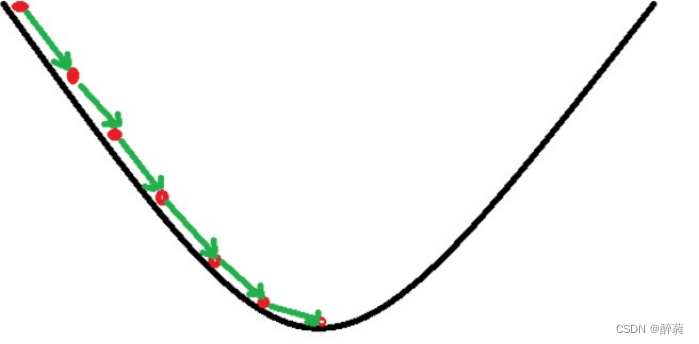如上图，假如为山的纵切面，那每次下山一小步，经过N次后你便可以到达山底。

# 二、数学解释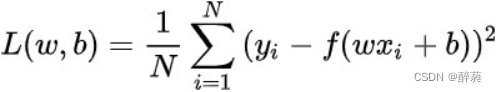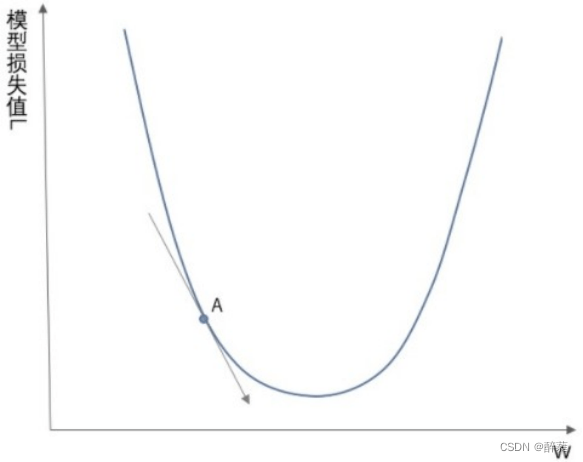梯度下降其实就是我们学习人工智能算法的一个基本算法，比如我们小学开始接触数学时候我们学习  加减乘除，最基本的算法

## 1、梯度

一阶函数里梯度就是表示某一函数在该点处的方向导数沿 着该方向取得较大值，即函数在*当前位置的导数*。如果函数为一元函数，梯度就是该函数的导数。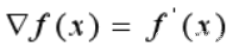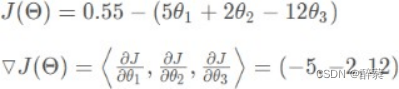我们可以看到，梯度就是分别对每个变量进行微分，然后用逗号分割开，梯度是用<>包括起来，说明梯度其实一个向量。向量有方向，梯度的方向就指出了函数在给定点的上升最快的  方向。

再看刚才的例子，我们需要到达山底，就需要在每一步观测到此时最  陡峭的地方，梯度就恰巧告诉了我们这个方向。梯度的方向是函数在给定点上升最快的方向那么梯度  的反方向就是函数在给定点下降最快的方向, 这正是我们所需要的 。所以我们只要沿着梯度的方向一直走，就能走到局部的最低点！## 2、核心公式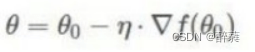## 3、步长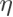（学习率）

前面一直讨论如何下山最快和如何用数学方法来解决下山最快和下山的方向，那么还忽视了一个问题，就是下山的步子。

我们可以通过来控制每一步走的距离，步长太大走的就容易偏离路线，其实就是不要走太快，错过了最低点。同时也要保证不要走的太慢，导致太阳下山了，还没有走到山下。所以的选择在梯度下降法中往往是很重要的！不能太大也不能太小，太小的话，可能导致迟迟走不到最低点，太大的话，会导致错过最低点！

## 4、梯度下降法的一般步骤1、给定待优化连续可微分的函数J（θ），学习率或步长，以及一组初始值（真实值）
2、计算待优化函数梯度
3、更新迭代
4、再次计算新的梯度
5、计算向量的模来判断是否需要终止循环

## 5、一元函数梯度下降实例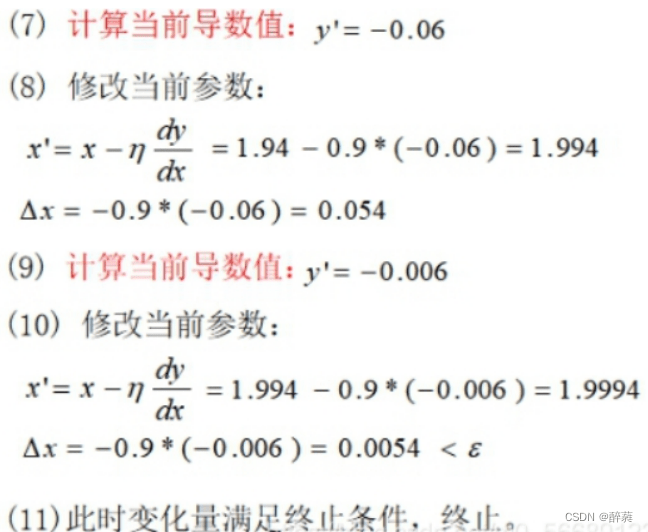代码段

``````#f(x)=x^2
import numpy as np
#定义原函数f(x)=x^2
def f(x):
return np.power(x, 2)
#定义函数求导公式1
def d_f_1(x):
return 2.0 * x
#定义函数求导公式2
def d_f_2(f, x, delta=1e-4):
return (f(x+delta) - f(x-delta)) / (2 * delta)
xs = np.arange(-10, 11)# 限制自变量x的范围
plt.plot(xs, f(xs))#绘图
plt.show()
learning_rate = 0.1# 学习率（步长）
max_loop = 30# 迭代次数
x_init = 10.0# x初始值
x = x_init
lr = 0.01# ε值，不过我们下面用的是迭代次数限制
for i in range(max_loop):
# d_f_x = d_f_1(x)
d_f_x = d_f_2(f, x)
x = x - learning_rate * d_f_x
print(x)

print('initial x =', x_init)
print('arg min f(x) of x =', x)
print('f(x) =', f(x))``````
``````//能做，但不建议使用
#include "stdio.h"

double fun(double x){       //定义初始函数
return x*x/2.0 - 2*x;
}

double der_fun(double x){       //求函数的导数
return x - 2.0;
}

int main(){
double length,accuracy;     //定义步长，精确度
double x0,x1;      //定义初始位置
printf("请输入步长,精确度及其初始位置：");
scanf("%lf %lf %lf",&length,&accuracy,&x0);
while(length*(-1)*der_fun(x0) > accuracy){
x0 = x0 - length*der_fun(x0);
}
printf("x = %.5lf\ny = %.5lf",x0,fun(x0));
return 0;

}
``````